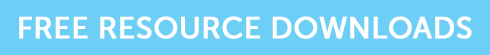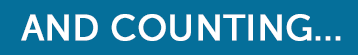The Greek Alphabet – Alpha, Beta and All That Jazz

# The Greek Alphabet – Alpha, Beta and All That Jazz

Written by Tina Winter

Back in the 1950s and 60s, an American academic by the name of Harry Markowitz carried out some theoretical studies on investment risk which we now know as Modern Portfolio Theory – this led to the Capital Asset Pricing Model (CAPM) which is still the cornerstone of modern investment theory.The CAPM says that not all portfolios have the same risk – a key factor to understanding the CAPM is beta (β), the sensitivity to market risk of an investment or portfolio. The average beta of shares in the market in 1.0, and a share with a beta of 1.0 will move exactly in line with the market – an index tracking fund would aim to have a beta as close to 1.0 as possible.

Cash has a beta of 0 or thereabouts, as its return is not linked to the market. An investment with a beta between 0 and 1 should rise (and fall) more slowly than the market, and the higher the beta, the greater the volatility of the share due to market moves. You won’t be expected to calculate beta in your exams but may be given a figure for it to use in calculations. Beta will always be a positive number.

The expected return on an investment equals the risk-free return plus the expected return on the market less the risk free return, both multiplied by the beta of the investment.

As an example, assume risk free return 3%, expected market return 6%, beta 1.2

Expected return = 3 + 1.2 (6 – 3)

= 3 + (1.2 x 3)

=  6.6%

So remember: Beta less than 1, less risk than the market, beta more than 1, more risk than the market

Alpha (α) is a measure of return that is independent of the underlying market performance – it’s a measure of an investment manager’s stock picking skill. Alpha can be a positive or negative number – positive alpha means a return greater than the market, negative alpha means a return less than the market. It’s calculated as follows:

Actual return – (risk free rate + beta x (market return – risk free rate))

So using the same figures as above for risk free return, market return and beta, and an actual return of 8%

Alpha = 8 – (3 + 1.2 (6 -3) = 8 – (3 + 3.6) = 8 – 6.6 = 1.4

So remember: Alpha positive good, Alpha negative bad!